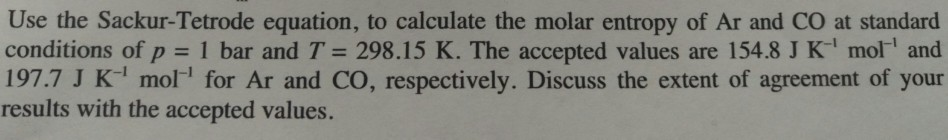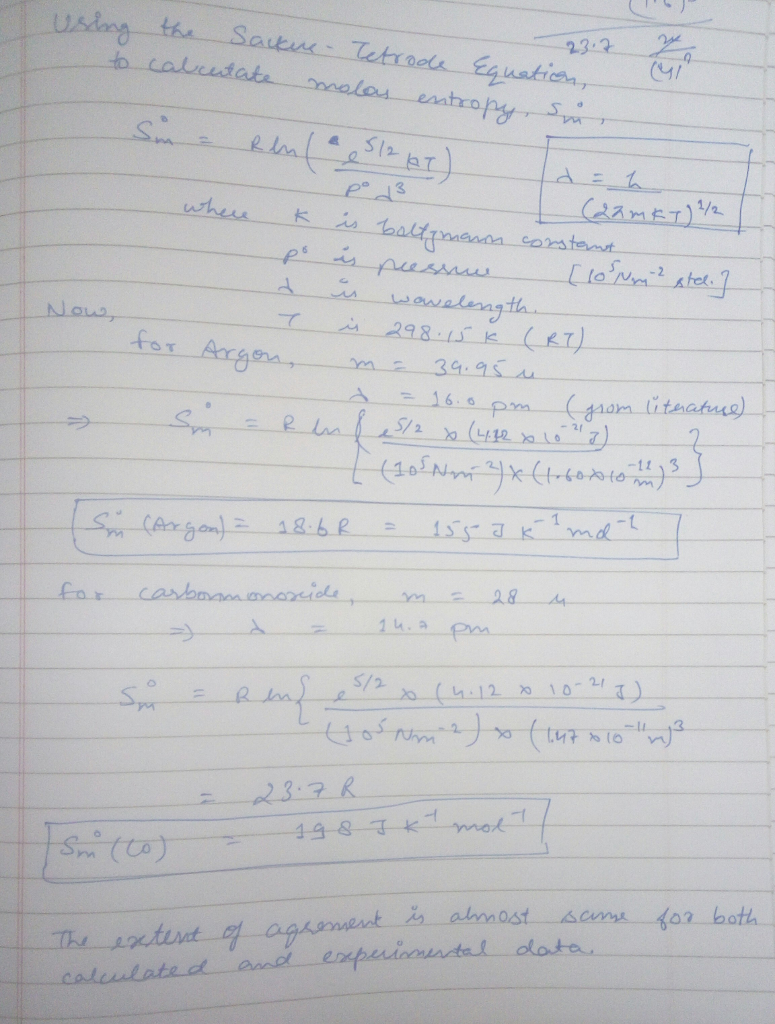# Question & Answer: Use the Sackur-Tetrode equation, to calculate the molar entropy of Ar and CO at standard…..Use the Sackur-Tetrode equation, to calculate the molar entropy of Ar and CO at standard conditions of p = 1 bar and T = 298.15 K. The accepted values are 154.8 J K^-1 mol^-1 and 197.7 J K^-1 mol for Ar and CO, respectively. Discuss the extent of agreement of your results with the accepted values.